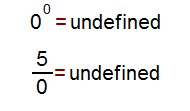# Basic Algebra

Using MatDeck to do numerical and algebraic calculations is very simple. Just enter the expression and place an equal sign at the end of the line, like in the examples below.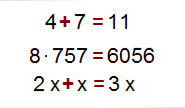Every line can be modified at any time: change any of values in above lines and the result will automatically change. We will do a few more calculations to illustrate an example. Create a power function by using Shift + 6 key combination.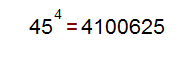To create a fraction node, use Alt + / key combination, or just / to do a division, without the visual creation of a fraction.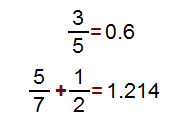To enter a square root of a number use the sqrt function: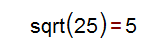MatDeck has a wide range of constants built in, that you can enter by typing the character c before a constant name. For example, if you want to enter π, you should type cpi.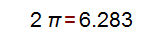To enter a natural exponential function ex type exp.For the absolute value function | | type abs, and the absolute value node will appear.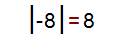For the natural logarithm ln( ) use the log function.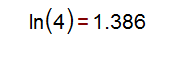For trigonometric function cosine, use the cos function, and if you want an inverse of cosine function use the acos function.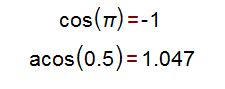Just a quick note: if you try to calculate a value that can’t be calculated, you will get an undefined response.